# Pandas - Add New Columns to DataFramesTo add new columns to dataframes with Pandas we have a couple of options depending on how simple or complex the calculations are for the new columns.

## Simple Method

The simple method involves us declaring the new column name and the value or calculation to use. This can be a constant of any pandas data type or a value calculated from other columns in the data frame.

df[new_column_name] = column_A + column_B

## Pandas Apply Function

For more complex column creation such as creating columns using functions, we can use the apply operation.

df[new_column_name] = df.apply(function_name, axis=1)

When we use the apply function and the axis=1 parameter we effectively pass each row of a DataFrame into the function that we declare in the parameters. If we build a custom function then we can use any combination of existing columns to create a new column and the logic inside the function can be as complex as required.

## Pandas Apply with Lambda

As an extension to the apply method we can also use Python’s lambda operation in place of a regular function as we can in any Python script. This is especially useful if the logic in our function only requires the use of 1 existing column to calculate value in our new column.

df[new_columns_name] = df[column_A].apply(lambda x: 1 if x >= 0 else 0)

In our data frame we have information about what was ordered and about the different costs and discounts associated with each order and product but a lot of the key financial and operational metrics are missing such as:

• Promotional Discount Value
• Gross Sales Value (Product price minus discount value)
• Net Sales Value (Gross sales value after returns)
• Net Products Sold
• Profit

Let’s create some new columns that calculate these metrics.

#### Discount Value

We have the discount rate but we don’t know what that is in monetary terms. Let’s create a new column called Discount_Value that gives us this:

df['Discount_Value'] = df['Retail_Price'] * df['Discount']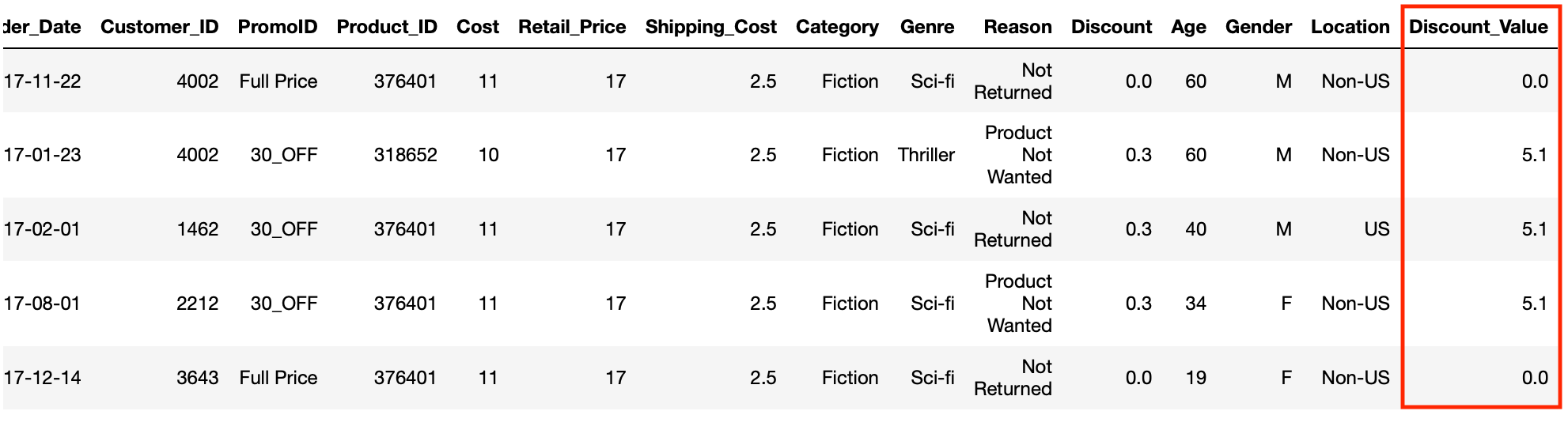#### Gross Sales Value

Now that we know the discount value that was applied to each order, we can calculate the gross sale value for each product that was ordered. This is the money the business received from the customer and the calculation needed here is simply the retail price minus the discount value.

df['Gross_Sale_Value'] = df['Retail_Price'] - df['Discount_Value']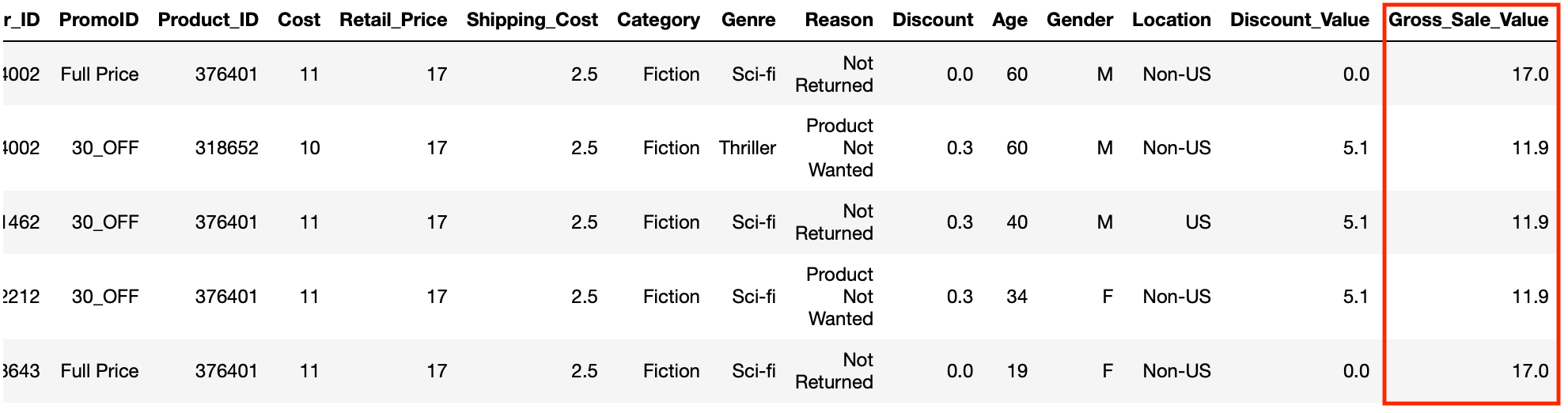#### Net Sales Value

This gives us some important sales information but doesn’t give us the full picture as we also need to know the net sales value which is the sales value once returns have been accounted for. In other words if a customer has kept the product then the net sales value is equal to the gross sales value but if the product was returned then the net sales value is zero as the business would have refunded the sale value back to the customer.

Whenever a customer kept a product the reason column contains a “Not Returned” string.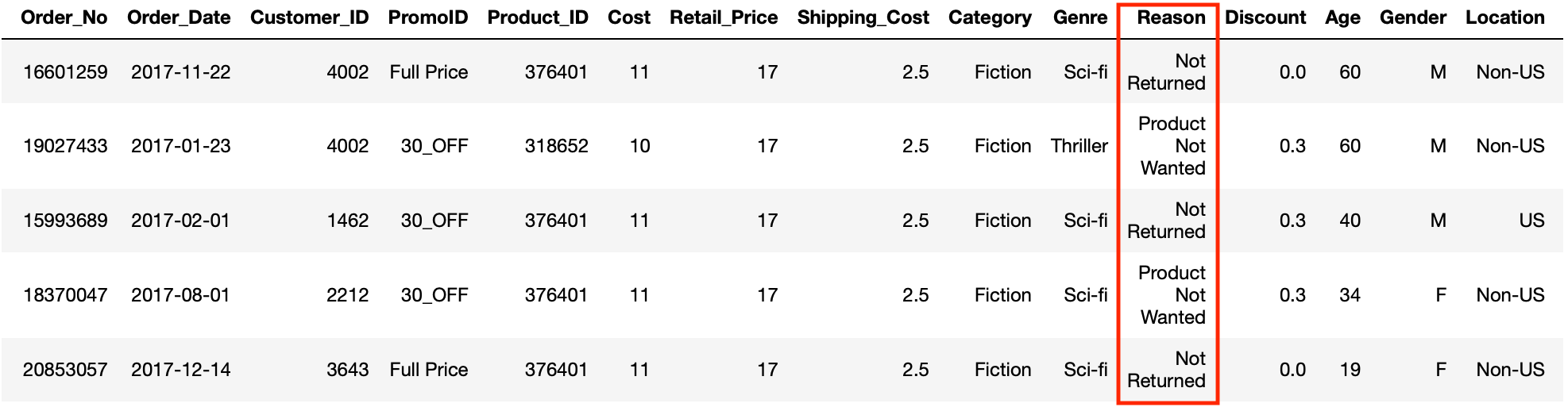So to get the net sales value we are going to have to define a function that returns a zero when the reason columns is equal to “Not Returned” but otherwise returns the gross sales value.

def get_net_sale_value(df_row):
if df_row['Reason'] == 'Not Returned':
net_sale_value = df_row['Gross_Sale_Value']
else:
net_sale_value = 0
return net_sale_value

Finally we use the apply operation to pass each row of our data frame to the function for it derive and then return the net sales value.

df['Net_Sale_Value'] = df.apply(get_net_sale_value, axis=1)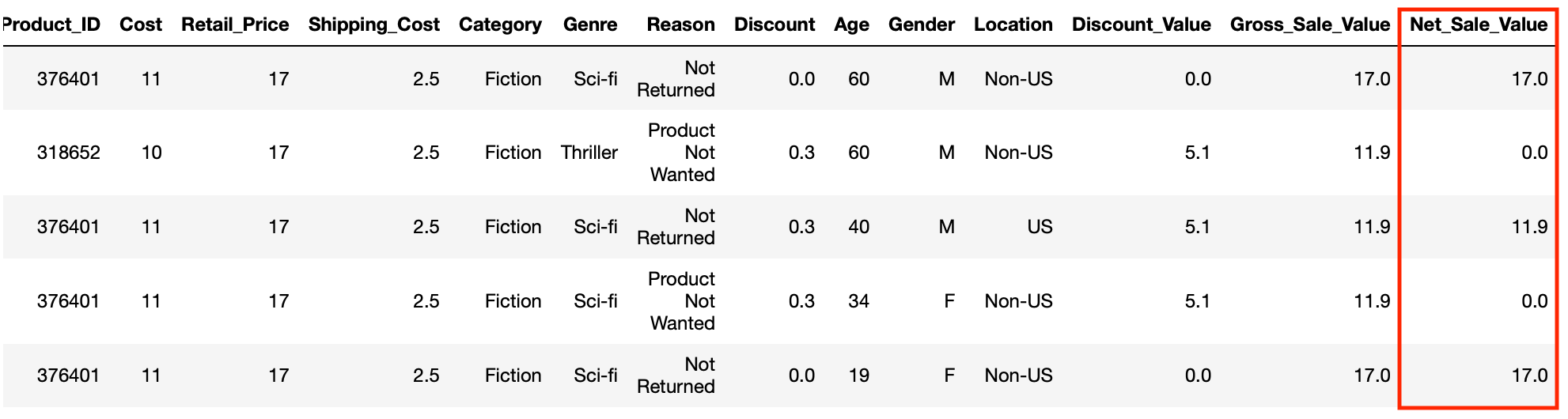#### Net Units Sold

We can use similar logic to the function we created to calculate net sales value to create a column that tells us the number of net units units sold (the number of units after returns have been subtracted). As we only have one product for each row this will either be a 1 or 0 but having this column will allow us to sum the net number of products sold when we aggregate our data. Let’s use lambda to create this column:

df['Net_Units_Sold'] = df['Reason'].apply(lambda x: 1 if x == 'Not Returned' else 0)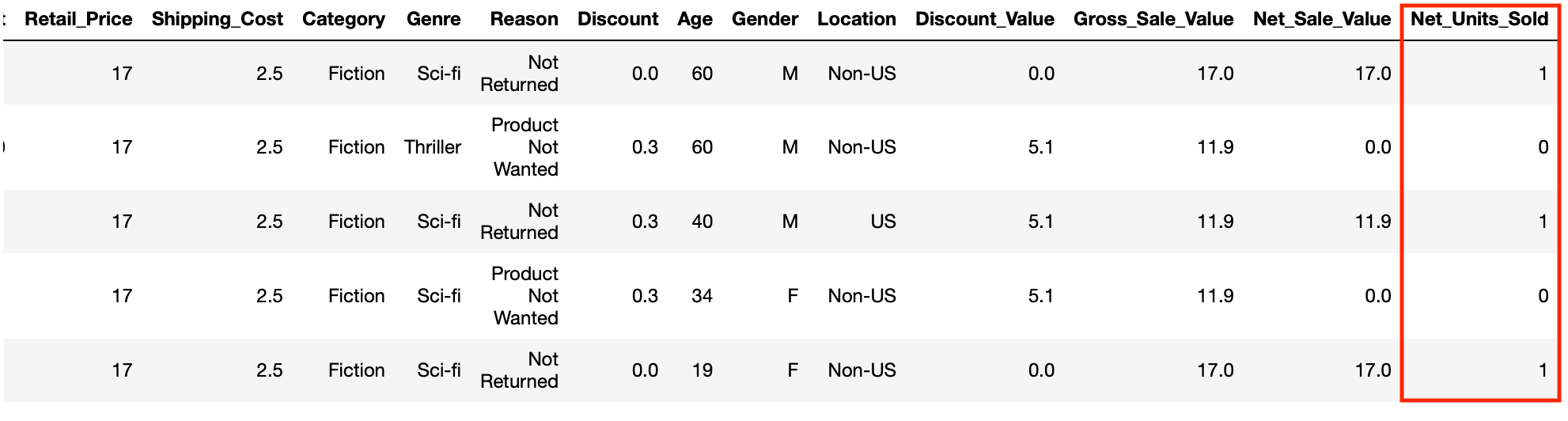## Exercises

For our final final few columns we need to know some information about how much profit (sale value after costs to the business has been calculated) was made for each product the business sold and how many products were returned.

1. Create a column called “Profit” that contains the net profit for each product sold which is Gross Sales Value minus Cost and then multiplied by the Net Units Sold
2. Create a column called “Profit_Margin” that tells us the percentage of the sale value that the business keeps as profit. The calculation for this should be the profit divided by the gross sales value
3. Finally let’s create a column that contains the number of units returned for each row. Hint: You can use a similar method to that used in creating Net_Units_Sold column

## Solutions

#### Exercise 1

df['Profit'] = (df['Gross_Sale_Value'] - df['Cost']) * df['Net_Units_Sold']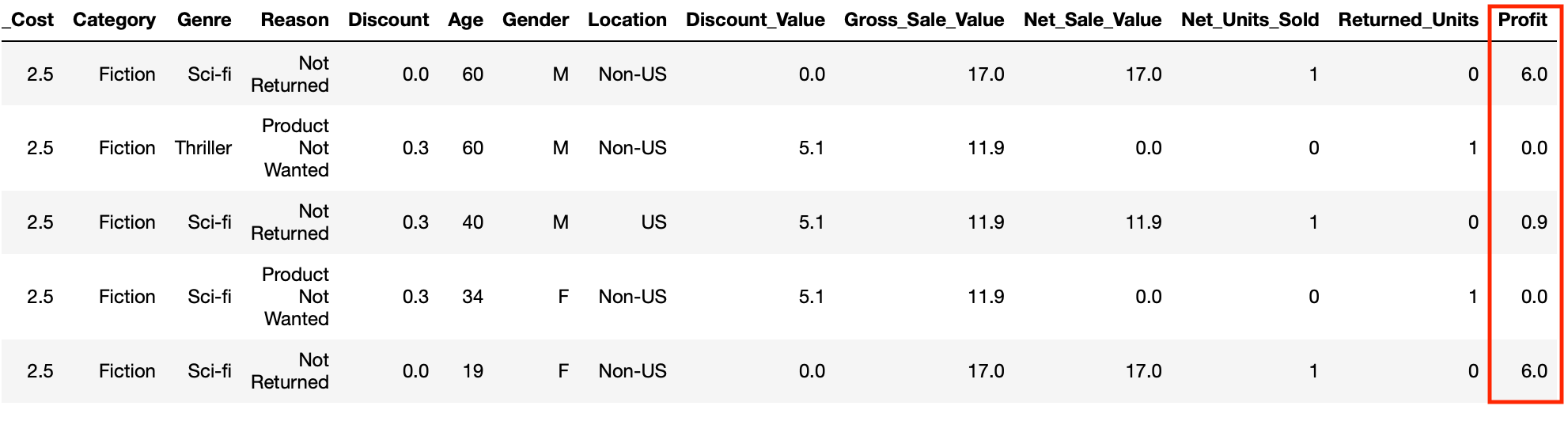Note: We need to multiply by net units sold to make sure that we see zero profit for returned products. We could have equally used the apply method and a function to more explicitly calculate this.

#### Exercise 2

df['Profit_Margin'] = df['Profit'] / df['Gross_Sale_Value']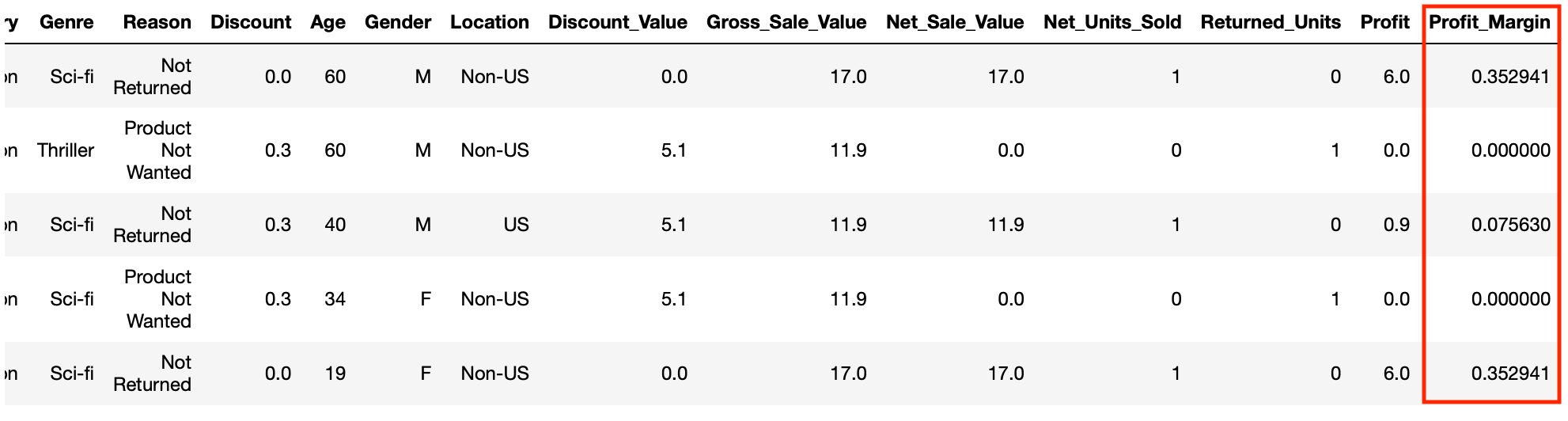#### Exercise 3

df['Returned_Units'] = df['Reason'].apply(lambda x: 0 if x == 'Not Returned' else 1)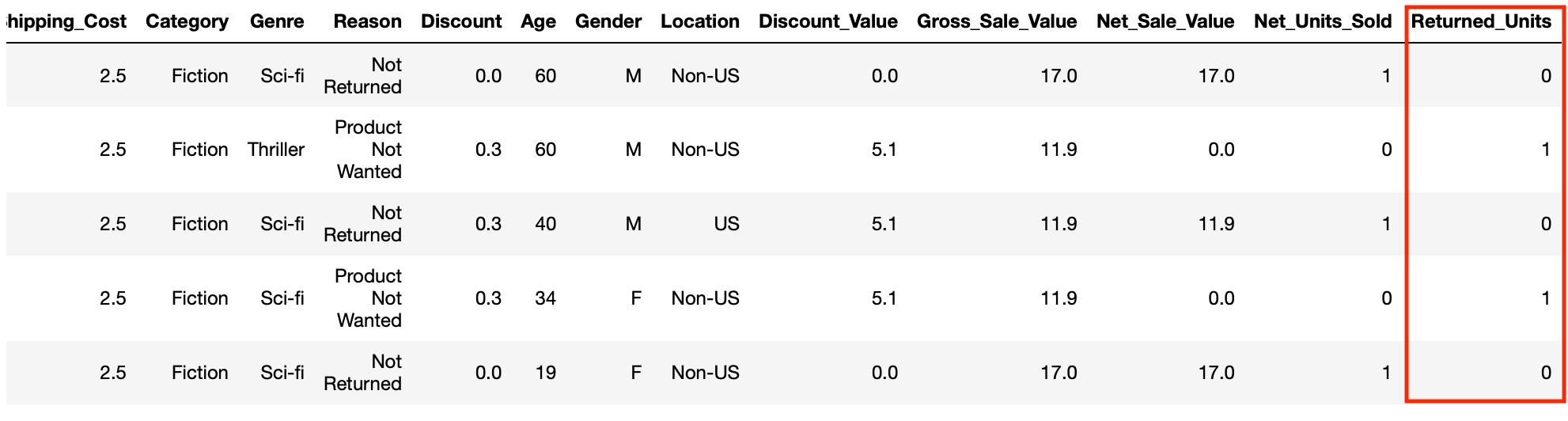Next